# How to Calculate a Ratio in Excel

This tutorial will show you how to compare two numbers and calculate their ratio, such as 5:7 or 8:3.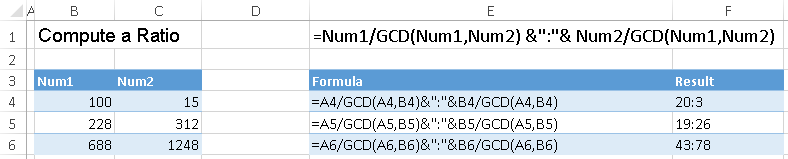## Calculate a Ratio – The GCD Method

The GCD Function is used to calculate the greatest common denominator between two or more values. We can use GCD to then create our ratios.

To use the GCD Function, you may need to enable the Data Analysis Toolpak. To check if you will need to enable the Data Analysis Toolpak, type =gcd( into an Excel cell. If you see the GCD function inputs appear: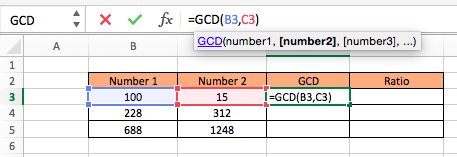For Mac
• Click on Tools. (Located at the very top where you have File and Edit.)
• A small pop tab should appear. Be sure that Analysis ToolPak is selected.
• Select OK.
• The ToolPak should now be available in the Data tab with the title “Data Analysis.” If you do not see it, you may need to reload your page and check again.

For PC
• Click the File button
• Find and select Options. (It is located near the bottom.)
• On the left side click Add-Ins
• Under Inactive Applications find and select Analysis ToolPak
• Click Go
• A new window titled “Add-Ins” should appear. Select Analysis ToolPak.
• Click OK
• The ToolPak should now be available in the Data tab with the title “Data Analysis.”

### Using the GCD Function

The GCD Function is extremely easy to use. Simply type =GCD( followed by the numbers that you wish to find the greatest common divisor of (separated by commas).

=GCD(Number 1, Number 2)

For example, our first row would be calculated as: =GCD(B3,C3) with the answer being 5.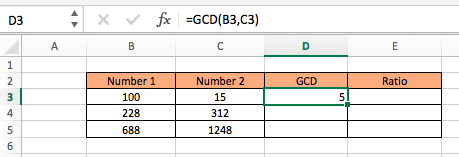Once you understand how GCD works you can use the function to find your ratios by dividing each of the two numbers by their GCD and separating the answers with a colon “:”.Keep in mind that the answer will be a text value.

The formula for the ratio is:

= Num1/GCD(Num1, Num2) & “:” & Num2/GCD(Num1, Num2)

This complicated formula is basically saying,

= (Number of times Number 1 contains GCD) & “:” & (Number of times Number 2 contains GCD)

In our example the formula would be written as:

=B3/GCD(B3,C3) & ”:” & C3/GCD(B3,C3)

Which results in:
=100/5 & “:” & 15/5
= 20 & “:” & 3
= 20:3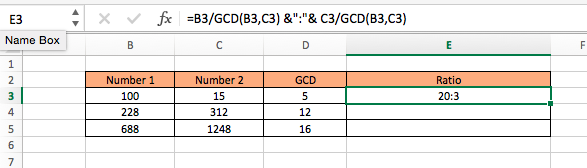## Calculate a Ratio – The Text & Substitution Method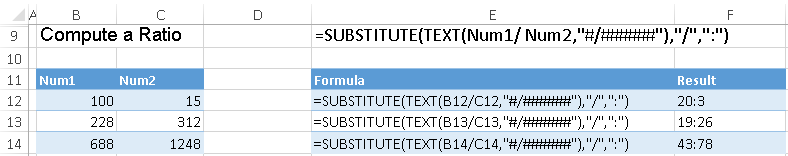Another method to calculate ratios is the Text & Substitution Method. This method does not require the Analysis ToolPak to be installed.

The Text Function allows you to display an answer as a text value. In our case it will divide Number 1 by Number 2, but instead of producing a decimal value the answer will be displayed as a fraction, such as 5/4 or 9/2.

The formula for the fraction is:

=TEXT(Number 1/Number 2, “#/######”)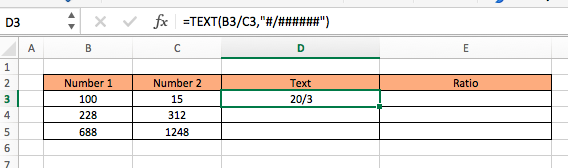The Text Function tells Excel to display the answer in the form “num1/num2” The extra ##### in the Text formula tells Excel to display the largest fraction. If you only specify #/# you may receive a rounded answer because Excel will display a fraction with only one digit for the numerator and denominator.

To display the fraction in the desired ratio format you will have to substitute the slash with a colon. This will involve using the Substitution Function.

=SUBSTITUTE(TEXT(Num1/Num2, “#/######”),”/”,”:”)

In our example this would be written as:
=SUBSTITUTE(TEXT(B3/C3, “#/######”),”/”,”:”)

This results in:
=SUBSTITUTE(TEXT(100/15, “#/######”),”/”,”:”)
= 20:3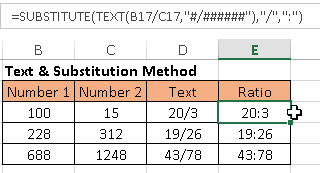This tutorial will teach you how to set up and calculate a running total
This tutorial explains how to count the number of cells containing numbers that fall
This tutorial covers how to calculate age in Excel. Calculate Exact Age To calculate the
This tutorial will demonstrate various ways to calculate compound interest in Microsoft Excel. What
This tutorial covers how to add entire rows or columns in Excel The Sum